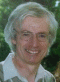Websense is a global leader in internet filtering and web security solutions to help companies productively use the internet. Unfortunately it had been blocking the ExcelCalcs site due it use of words like ‘Free software’ and ‘downloads’. I appealed to the Websense and I am pleased to report that they have removed the block. I imagine that many people working for large organisations have not been able to access the site because of this problem so if you have recommended the site to friends and colleagues please remind them to take another look by forwarding this email. Thanks.

Repository NewsI would like to make a special thank you to Doug Jenkins for his kind contribution to the ExcelCalcs repository of solved problems. Doug Jenkins is a Civil Engineering consultant based in Sydney Australia (Interactive Design Services Pty Ltd). His experience spans some thirty years and he has been involved with prestigious projects around the world. Doug has developed some very powerful Excel functions. Doug appears to prefer development of functions rather than calculations on the spreadsheet itself. If you have never written any macros in Excel his calculations serve as excellent examples you can easily follow.

New!Quartic2.xls
 Find the roots of polynominal equations with Purpose Excel Functions. Author Doug Jenkins. Quartic - ax^4 + bx^3 + cx^2 + dx +e = 0 =quartic(a, b, c, d, e, Output Index -1 to 5 (optional)) Cubic - ax^3 + bx^2 + cx + d = 0 =cubic(a, b, c, d, Output Index -1 to 4 (optional)) CubicCa - x^3 + bx^2 + cx + d = 0 =cubicC(a, b, c, d, Output Index -1 to 4 (optional)) Quadratic - ax^2 + bx + c = 0 =quadratic(a, b, c, Output Index 1to 3(optional))
New!SecProps.xls
 Excel custom functions for calculation of section properties from coordinates. Author Doug Jenkins.     Function AREA(ArrayOfCoordinates)     Function AX(ArrayOfCoordinates)     Function AY(ArrayOfCoordinates)     Function IXO(ArrayOfCoordinates)     Function IYO(ArrayOfCoordinates)     Function IXYO(ArrayOfCoordinates)     Function XBAR(ArrayOfCoordinates)     Function YBAR(ArrayOfCoordinates)     Function IXC(ArrayOfCoordinates)     Function IYC(ArrayOfCoordinates)     Function IXYC(ArrayOfCoordinates)     Function IU(ArrayOfCoordinates)     Function IV(ArrayOfCoordinates)     Function THETA(ArrayOfCoordinates)
Updated!Simple 1D conduction.xls
New!Doug's Maths Functions
 Useful maths functions from Doug Jenkins. Function IP: Finds the intersection points of two 2D lines or polylines; Function IPLC: Finds the intersection points of a 2D line and a circle; Function IPCC: Finds the intersection points of two circles; Function IPSSS: finds the 3D intersection points of three spheres; Function IPSS: finds the location and radius of the intersection circle of two spheres; Function RtoP, PtoR: Converts rectangular to polar coordinates and polar to rectangular; Function Rotate: Rotates 2D or 3D rectangular axes about any axis; Function Interp, Interp2, Loginterp, Loginterp2, Quadinterp: One way and 2 way linear, log interpolation and quadratic interpolation.
New!Mike Hadden's Excel and Maths Files.
Updated!PRYING9.xls
Updated!ENDPLMC9.xls
Updated!piping_design_info.xls